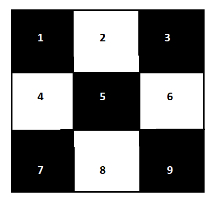Count squares with odd side length in Chessboard in C++

C++Server Side ProgrammingProgramming

Given a number size as input as dimension of size*size Chessboard. The goal is to find the number of squares that can be formed inside that board having odd lengths.

For Example

size=3

Output

Count of squares with odd side length in Chessboard are: 10

Explanation

All squares will be as shown : and 1 whole square of size 3x3.size=4

Output

Count of squares with odd side length in Chessboard are: 20

Explanation

there will be 16, 1X1 squares. And 4, 3X3 squares inside it.

Approach used in the below program is as follows

In this approach we will traverse from length of square as 1 to length as size. For each odd length we will add ( size−i−1)2 to the count.

• Take an integer size as input for Chessboard’s side.

• Function square_odd_length(int size) takes size and returns count of squares with odd side length in Chessboard.

• Take the initial count as 0.

• Traverse from i=1 to i=size increment by 2 for odd values of i.

• For each i take temp=size−i+1.

• At the end of the for loop return count as result.

Example

Live Demo

#include <bits/stdc++.h>
using namespace std;
int square_odd_length(int size){
int count = 0;
for (int i = 1; i <= size; i = i + 2){
int temp = size − i + 1;
count = count + (temp * temp);
}
return count;
}
int main(){
int size = 6;
cout<<"Count squares with odd side length in Chessboard are: "<<square_odd_length(size);
return 0;
}

Output

If we run the above code it will generate the following output −

Count squares with odd side length in Chessboard are: 56